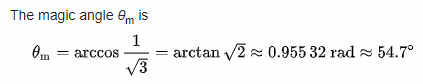# Isometric view from Top Viewport

Hi everyone!

I have been trying to rotate my model (any model actually) in order for it to be baked and be able to run Make2D directly from Top view.

According to this Wikipedia article, there are 2 rotations to achieve Isometric View,:

For example, with a cube, this is done by first looking straight towards one face. Next, the cube is rotated ±45° about the vertical axis, followed by a rotation of approximately 35.264° (precisely arcsin ​1⁄√3 or arctan ​1⁄√2, which is related to the Magic angle) about the horizontal axis.

But I must be missing something…

Isometric.gh (6.0 KB)

Use plane YZ instead of XZ for the second Rotate:

Isometric_2019Nov9a.gh (9.8 KB)

Hi Joseph, thank you.

Unfortunately I tried that too and it doesn’t seem to do it either

The angle here is supposed to be 120 degrees.

OK, your angle is wrong. I did a quick experiment and determined that the three lines you refer to (“101.54”), projected to World XY, become equal in length when the angle is ~54 degrees. As explained in the Wikipedia citation:Which is \text{arctan}(\sqrt{2}), not \text{arctan}\!\left(\frac{1}{\sqrt{2}}\right):

Isometric_2019Nov9b.gh (10.5 KB)

FYI, here is the “test jig” I rigged up; I was intending to measure the angles between these three lines, then realized that they would be of equal length which is more expedient to measure:

Isometric_2019Nov9aa.gh (16.6 KB)

Isometric views take me way back to mechanical drawing classes in high school, where X and Y lines were drawn at 30 degrees using a plastic triangle and T-square.

Thanks Joseph,

Hmm thats weird. Wikipedia explicitly says

approximately 35.264° (precisely arcsin ​1⁄√3 or arctan ​1⁄√2, which is related to the Magic angle.

Meaning it is related to 54.73 but not the one used to create an isometric.

Oh well, it seems to work, thank you.